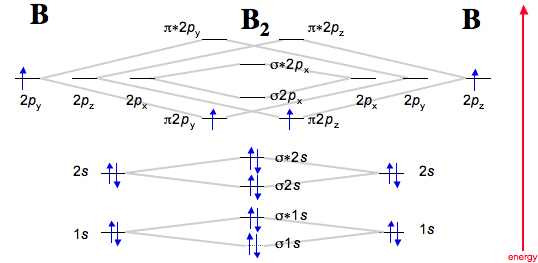# 5.7A: $$\pi$$-Bonding in $$CO_2$$

$$\newcommand{\vecs}{\overset { \rightharpoonup} {\mathbf{#1}} }$$ $$\newcommand{\vecd}{\overset{-\!-\!\rightharpoonup}{\vphantom{a}\smash {#1}}}$$$$\newcommand{\id}{\mathrm{id}}$$ $$\newcommand{\Span}{\mathrm{span}}$$ $$\newcommand{\kernel}{\mathrm{null}\,}$$ $$\newcommand{\range}{\mathrm{range}\,}$$ $$\newcommand{\RealPart}{\mathrm{Re}}$$ $$\newcommand{\ImaginaryPart}{\mathrm{Im}}$$ $$\newcommand{\Argument}{\mathrm{Arg}}$$ $$\newcommand{\norm}{\| #1 \|}$$ $$\newcommand{\inner}{\langle #1, #2 \rangle}$$ $$\newcommand{\Span}{\mathrm{span}}$$ $$\newcommand{\id}{\mathrm{id}}$$ $$\newcommand{\Span}{\mathrm{span}}$$ $$\newcommand{\kernel}{\mathrm{null}\,}$$ $$\newcommand{\range}{\mathrm{range}\,}$$ $$\newcommand{\RealPart}{\mathrm{Re}}$$ $$\newcommand{\ImaginaryPart}{\mathrm{Im}}$$ $$\newcommand{\Argument}{\mathrm{Arg}}$$ $$\newcommand{\norm}{\| #1 \|}$$ $$\newcommand{\inner}{\langle #1, #2 \rangle}$$ $$\newcommand{\Span}{\mathrm{span}}$$

Molecular Orbital (MO) theory is used by scientists to understand bonding in molecules. Carbon dioxide is a linear, centrosymmetric molecule with D∞h symmetry. Surrounded by two oxygens, carbon is the central atom. MO therory predicts π bond formation resulting from the interaction of C 2Px and y atomic orbitals with O fragment LGO(Ligand Group Orbitals).

## Introduction

Carbon dioxide is a well studied molecule. Carbon dioxide is a covalent compound comprised of three atoms, carbon surrounded by two oxygens. Both carbon and oxygen contain p orbitals that are able to interact based on symmetry compatability. Valence bond (VB) theory predicts four bonds for carbon and two for each oxygen. The bond order of each carbon-oxygen bond is 2. Carbon dioxide contains two double bonds. Each double bond is comprised of one sigma bond and one π bond. Carbon dioxide is an important reagent used in industry, is a cental aspect of our global carbon cycle and is the basis for climate change. Lastly, Molecular Orbital (MO) theory is a better tool to use than VB theory because it does not assume localization of electrons. MO theory is based on Linear Combinations of Atomic Orbitals (LCAO).

## MO Theory

Mo theory is used to predict bonding, anti-bonding and non-bonding orbitals. Anti-bonding orbitals are always higher in energy than corresonding bonding orbitals. Anti-bonding orbitals are usually indicated with an *. For example, σ*=sigma anti-bonding and σ=sigma bonding. Bonding can be thought of as atomic orbitals being in phase (constructive) and anti-bonding as being out of phase (destructive). Mo diagrams are graphical representations of orbital interactions based on symmetry compatability. Mo diagrams are based on individual atom electron configurations. For example, B=1s22s22p1. Boron has three valence electrons (highest n=principle quantum number in nS or nP) and 2 core electrons. Diatomic Boron (B2) has an MO diagram:http://www.meta-synthesis.com/webbook/39_diatomics/diatomics.html

Notice that each individual B atom has 3 valence(n=2) electrons. When combined to form molecular orbitals the bond order is 1: BO=1/2(Bonding-Anti).

## Carbon Dioxide MO diagram

The carbon dioxide MO diagram is based on a C atom and an O--O ligand fragment. Carbon has 2S and 2Px,y,z orbitals and the O--O fragment has 2S and 2Px,y,z orbitals that are involved in the formation of molecular orbitals. Since CO2 has D∞h symmetry the central atom's orbital symmetry lables can be obtained from the corresponding point group table: 2S=σg, 2Pzu and 2Px,yu. The LGO symmetry lables can be calculated using the point group table as well: Γσ=2σg + 2σu and Γπ=2πg + 2πu. The MO diagram for CO2 is more complicated than the diagram for B2. The follwing diagram fails to label orbital symmetries but the LGO 2Px,y particpate in the formation of π double bonds. The 2πg orbiatls are nonbonding because the C 2Px,y atomic orbitals are πu. The LGO 2Pz orbitals are involved in σ bonds.http://cnx.org/content/m32935/latest/

Ater the formation of σ bonds resulting from C 2S and 2Pz electrons with O 2S and 2Pz orbitals the remaining C 2Px and 2Py orbitals interact with the O LGO fragment. Qualitatively, πu(2Px,y) is dipected by the MO diagram as dumbbells overlaping in phase and πg(2Px,y)* as overlapping out of phase.

## References

1. Housecroft, C.E., and Sharpe, A.G., Inorganic Chemistry, 3rd edition, Pearson Education Limited, Edinburgh, 2008.
2. McQuarrie D.A., and Simon, J.D., Physical Chemistry A Molecular Approach, University Science Books, 1997.